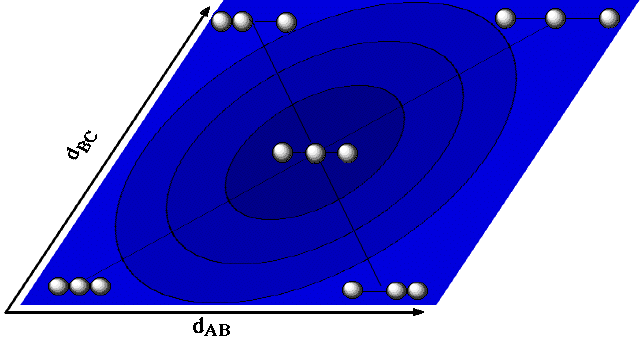Potential Energy Surface for Linear H3
(and why the axes are not at 90°)

from H. Eyring, H. Gershinowitz, and C.E. Sun, J. Chem. Phys., 3, 786 (1935)Why are the axes not at 90°? Because of the need to model kinetic energy as well as potential energy with the rolling ball. [In Chem 125 you are not responsible for this explanation, but here it is in case you're ever curious.]

 Consider a circular "well" on the PES (for a stable linear triatomic molecule like Lake Eyring or O=C=O) and think about the ball rolling in it. Rolling across the pit from bottom left to top right involves symmetrical stretching of both bonds simultaneously. To stretch both bonds by an amount x, both terminal atoms have to move by that distance. Rolling from top left to bottom right unsymmetrically stretches one bond while the other shrinks. Changing the bonds asymmetrically by an amount x might be easier (or harder) in terms of potential energy than changing them symmetrically (the shape of the well might be ellipsoidal instead of round as shown), but unsymmetrical vibration is certainly easier in terms of kinetic energy, because only one atom (the central one) has to move very much. There would be a lot more inertia opposing vibration in the symmetrical mode, where two atoms move a lot than, than in the asymmetrical mode, where only one does. So even if the two kinds of distortion involved the same changes in potential energy (circular well) vibration along the symmetrical direction should be slower. To be realistic the marble should roll more slowly for the symmetric vibration.How can a single rolling marble simulate the difference in inertia for symmetrical and unsymmetrical stretching of a triatomic molecule? If we decrease the angle between the dAB and dBC axes, the circular valley becomes ellipsoidal, elongated from bottom left to top right.Now the distance the marble has to cover to achieve a given change in bond distance and energy is greater for the symmetrical motion than for the unsymmetrical. Since the slope is more gentle, symmetrical vibration will be slower, as desired.

Pretty clever.

An angle of 60° does the trick for three atoms of equal mass (linear H3).

To test your mastery of this idea, think about why the angle between the axes should be even less than 60° for O=C=O, where the terminal atoms are heavier than the central one.